American Journal of Physical Chemistry
Volume 4, Issue 5, October 2015, Pages: 38-41

Solutions of Nonrelativistic Schrödinger Equation with Scarf II Plus Rosen-Morse II Potential via Ansaltz Method

Akaninyene D. Antia

Theoretical Physics Group, Department of Physics, Faculty of Science, University of Uyo, Uyo, Akwa Ibom State, Nigeria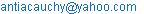(A. D. Antia)(A. D. Antia)

Akaninyene D. Antia. Solutions of Nonrelativistic Schrödinger Equation with Scarf II Plus Rosen-Morse II Potential via Ansaltz Method.American Journal of Physical Chemistry.Vol.4, No. 5, 2015, pp. 38-41. doi: 10.11648/j.ajpc.20150405.11

Abstract: We have solved the non-relativistic Schrödinger equation with Scarf II plus Rosen-Morse II potential analytically for arbitrary l-state by using the newly improved ansaltz for the wave function and adopting the modified approximation scheme to evaluate the centrifugal term. The bound state energy spectrum and the un-normalized wave function expressed in terms of Jacobi polynomial are also obtained. With this method, we have obtained a negative energy spectrum for the system.

Keywords: Non-Relativistic Schrödinger Equation, Scarf II Potential, Rosen-Morse II Potential, Bound State,
Wave Function

1. Introduction

The exact and analytical solutions of the non-relativistic and relativistic equations are of great importance in physics. The studies of these equations for some special potentials of interest have been successful over the years [1-10]. In non-relativistic quantum mechanics, it is well known that the exact solutions of Schrödinger equation are possible only for a few set of quantum systems.

However, when arbitrary angular momentum quantum numberis present, one can only solve the Schrödinger equation approximately using suitable approximation schemes . Some of such approximations include conventional approximation scheme proposed by Greene and Aldrich , improved approximation scheme by Jia et al., elegant approximation scheme  etc. These approximations are used to deal with the centrifugal term or potential barrier arising from the problem.

In solving non-relativistic or relativistic wave equation whether for central or noncentral potential, various methods are used. These methods include asymptotic iteration method (AIM) , supersymmetric quantum mechanics (SUSYQM) , shifted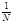expression , factorization method [18, 19], Nikiforov-Uvarov (NU)  and others [21, 22]. Also in recent times, much attention has been paid to factorization method. This method gives accurate analytical solutions for many differential equations that are important in applications to many problems in physics, such as the equation of Hermit, Lequerre, Legendre, Bessel and Jacobi.

However, the factorization method gives a complete analytical solution of Schrödinger equation for Woods-Saxon, Poschl-Teller and harmonics potentials. Recently, factorization method has been used to find the exact solutions of Schrödinger equation for inverted Woods-Saxon and Manning-Rosen potential . Satisfied by the factorization method through comparisons with other methods, we are tempted to solve the time-independent Schrödinger equation for Scarf II plus Rosen-Morse II potential. The main aim of this paper is to obtain approximate solutions of the Schrödinger equation with Scarf II plus Rosen-Morse II potential using an ansaltz to the wave function together with an approximation scheme to evaluate the centrifugal term.

2. Scarf II Plus Rosen-Morse Potential

The potential for this system is given as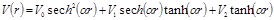(1)

where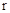is the radius of the nuclei,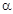is a parameter, and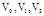are potential depths of the nuclear. This potential plays important role in many different fields of physics such as chemical and molecular physics. It could be used to describe nucleon-nucleon interactions, meson-meson interaction and also in various branches of nuclear physics and quantum chemistry.

3. Factorization Method

In spherical coordinate the Schrödinger equation with potential of Eq. (1) can be written as follows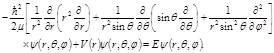(2)

The total wave function in Eq. (2) can be defined as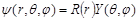(3)

and by decomposing the spherical wave function in Eq. (2) using Eq. (3) we obtain the following equations:(4)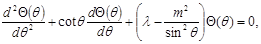(5)(6)

whereand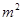are the separation constants.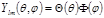are the solutions of Eqs. (5) and (6).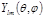are the spherical harmonics and their solutions are well known . Equation (4) is the radial part of Schrödinger equation which is subject for discussion in the preceding section.

4. Solutions of the Radial Schrödinger Equation and Energy Eigenvalues

The radial part of the Schrödinger equation from Eq.(4) is obtained as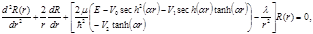(7)

Equation (7) in its present form has no analytical solution for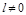, to to solve it an approximation has to be made for the centrifugal term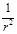. We write the centrifugal term in Eq.(7) as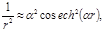(8)

Substituting Eq.(8). Into Eq. (7), we have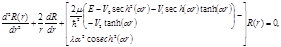(9)

Now using the common ansaltz,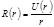, Eq.(9) could be transformed into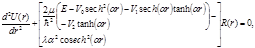(10)

Where the last term provides a centrifugal potential, which together with third, fourth and fifth terms comprise the effective potential. By using a new variable, we can rewrite Eq. (10) as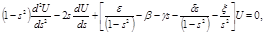(11)

where the following dimensionless quantities have been defined as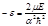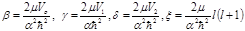(12)

Equation (12) is the well known associated Jacobi differential equation. In order to solve Eq. (11) explicitly, we invoke the new ansaltz for the wave function of the form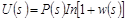(13)

Where P(s) is the associated Jacobi polynomial satisfying Eq.(11). Now substituting Eq.(13) into Eq.(11) and after a little algebraic, we obtain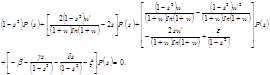(14)

Comparing Eq. (14) with the standard associated Jacobi differential equation [26, 27]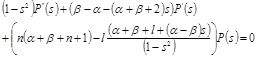(15)

We obtain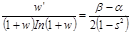(16)

Equation (16) is obtained by comparing Eq.(14) with Eq.(15) and integrating Eq.(16) and solving by partial fraction, we have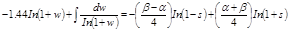(17)

Integrating the second term on the left hand side of Eq.(16), we have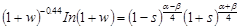(18)

Taylor expanding the first and second terms on the left hand side of Eq.(18) to first order, we have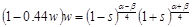(19)

Solving Eq.(19) explicitly, we obtain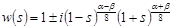(20)

In obtaining Eq.(20) from Eq.(19), we have assumed that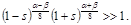Based on this assertion we see that the wave function of Eq.(20) is controlled by the imaginary part of the wave function. Thus, we write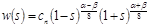(21)

Where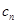is the normalization constant. Equation (14) obtained from the new ansaltz of Eq.(13) can be considered as the associated Jacobi differential equation. The associated Jacobi polynomial with variable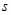from Eq.(15) can be written as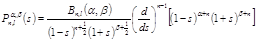(22)

Where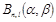is the normalization constant andare non-negative integers defined in the interval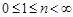.

The wave function can be obtained from Eq.(13) as(23)

Thus, the final form of the radial wave function can be written in terms of the Jacobi polynomials resulting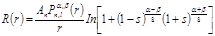(24)

By Taylor expanding the terms in the logarithm to first order, we get(25)

Using the transformation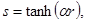Eq.(25) becomes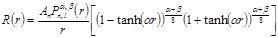(26)

Where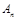is the new normalization constant. Further comparison of Eq.(14) with Eq.(15) gives the energy spectrum for the Scarf II plus Rosen-Morse II potential as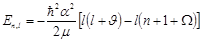(27)

Where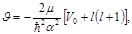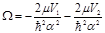.

5. Conclusion

In this paper, we have obtained the energy spectrum and wave function of Scarf II plus Rosen-Morse II potential for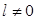analytically using an ansaltz for the wave function. The un-normalized wave function obtained is expressed in terms of Jacobi polynomial. In obtaining our result, we have invoked an approximation scheme to deal with the centrifugal term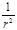. The results will have many applications in nuclear physics especially in chemical and molecular physics and the recently reported result of neutron-proton pairs in heavy nuclei using perturbation theory  where it could be used in describing nuclei interactions. This method can be applied to the investigation of other physical systems.

References

1. L. Z. Yi, Y. F. Diao, J. Y. Liu, C. S. Jia. Bound state of the Klein-Gordon equation with vector and scalar Rosen-Morse type potentials. Physics Letter A, Vol. 333, 212, 2004.
2. A. N. Ikot. Analytical solutions of Schrodinger equation with generalized hyperbolic potential using Nikiforov-Uvarov method. African Review of Physics, Vol. 6, No. 0026, pp. 221-228, 2011.
3. A. D. Antia, I. E. Essien, E. B. Umoren, C. C. Eze. Approximate solutions of the non-relativistic Schrodinger equation with inversely quadratic Yukawa plus Mobius square potential via parametric Nikiforov-Uvarov method. Advances in Physics Theories and Applications, Vol. 44, pp.1-13, 2015.
4. A. D. Antia, I. O. Akpan, A. O. Akankpo.Relativistic treatment of spinless particles subject to modified Scarf II potential. International Journal of High Energy Physics, Vol. 2, No.4, pp.50-55, 2015.
5. A. D. Antia, A. N. Ikot, E. E. Ituen, I. O. Akpan. Bound state solutions of the Klein-Gordon equation for deformed Hulthen potential with position dependent mass. Sri Lankan Journal of Physics, Vol. 13, No. 1,pp. 27-40, 2012.
6. H. Hassanabadi, S. Zarrinkamar and A. A. Rajabi. Exact solutions of D-dimensional Schrodinger equation for energy-dependent potential by Nikiforov-Uvarov method. Communications in Theoretical Physics, Vol. 55, 541, 2011.
7. W. C. Qiang and S. H. Dong. The Manning-Rosen potential studied by a new approximation scheme to the centrifugal term. Physica Scripta, Vol. 79, 045004, 2009.
8. A. D. Antia, A. N. Ikot, H. Hassanabadi and E. Maghsoodi. Bound state solutions of Klein-Gordon equation with Mobius square plus Yukawa potentials. Indian Journal of Physics, Vol. 87, No. 11, pp.1133-1139, 2013.
9. G. F. Wei, C. Y. Long and S. H. Dong. The scattering of the Manning-Rosen potential with centrifugal term. Physica Scripta, Vol. 77, 035001, 2008.
10. S. Dong, S. H. Garcia-Ravalo and S. H. Dong. Quantization rule solution to the Hulthen potential in arbitraryl-state. Physica Scripta, Vol. 76, 393, 2007.
11. J. Lu. Approximate spin and pseudospin solutions of the Dirac equation. Physica Scripta, Vol. 72, 349, 2005.
12. R. L. Greene and C. Aldrich. Variational wave functions for a screened coulomb potential. Physical Revision A, Vol. 14, 2363, 1976.
13. C. S. Jia, T. Chen and L. G. Cui. Approximate analytical solutions of the Dirac equation with the generalized Poschl-Teller potential including the pseudo-spin centrifugal term. Physics Letter A, Vol. 373, pp.1621-1626, 2009.
14. E. H. Hill. The theory of vector spherical harmonics. American Journal of Physics, Vol. 22, pp.211-221, 1954.
15. F. Yasuk and M. K. Bahar. Approximate solutions of the Dirac equation with position-dependent mass for the Hulthen potential by the asymptotic iteration method. Physica Scripta, Vol. 85, 045004, 2012.
16. H. Hassanabadi, E. Maghsoodi and S. Zarrinkamar. Relativistic symmetries of Dirac equation and the Tietz potential. European Physics Journal Plus, Vol. 127, 31, 2012.
17. M. Bag, M. M. Panja, R. Dutt and Y. P. Varshni. Modified shifted large-N approach to the Morse potential. Physical Review A, Vol. 46, pp. 6059-6065, 1992.
18. A. D. Antia, E. E. Ituen, H. P. Obong and C. N. Isonguyo. Analytical solutions of the modified coulomb potential using the factorization method. International Journal Recent advances in Physics, Vol. 4, No. 1, pp.55-65, 2015.
19. S. M. Ikhdair and R. Sever. Exact polynomial eigen solution of Schrodinger for pseudo harmonics potential. International Journal of Modern Physics, Vol.19, pp.221-229, 2008.
20. A. F. Nikiforov and V. B. Uvarov. Special Functions of mathematical Physics (Basel: Birkhauser), 1988.
21. A. N. Ikot. Solutions of the Klein-Gordon equation with equal scalar and vector modified Hylleraas plus exponential Rosen-Morse potential. Chinese Physics Letter, Vol. 29, 060307, 2012.
22. H. Hassanabadi, B. H. Yazarlo, S. Zarrinkamar and A. A. Rajabi. Duff-Kemmer-Petiau equation under a scalar Coulomb interaction. Physics Revision C, Vol. 84, 064003, 2011.
23. A. N. Ikot, L. E. Akpabio and E. B. Umoren. Exact solution of Schrodinger equation with inverted Woods-Saxon and Manning-Rosen potential. Journal of Scientific Research, Vol. 3, No. 1, pp. 25-33, 2011.
24.  L. D. Landau and E. M. Lifshitz. Quantum Mechanics, Non-relativistic Theory (Canada: Pergamon), 1977.
25. L. I. Schiff. Quantum Mechanics (New York: McGraw Hill), 1955.
26. A. D. Polyanin and A. V. Manzhirov. Handbook of integrals equation(CRC press Boca Rotan), doi: 10.1201/9781420050066, 1998.
27. M. R. Pahlavai, J. Sadeghi and M. Ghezelbash. Applied Science, Vol. 11, 106, 2009.
28. K. M. Khanna, G. F. Kanyeki, S. K. Rotich, P. K. Torongey and S. E. Ameka. Indian Journal Pure and Applied Physics, Vol. 48, 7, 2010.

 Contents 1. 2. 3. 4. 5.
Article ToolsAbstractPDF(166K)